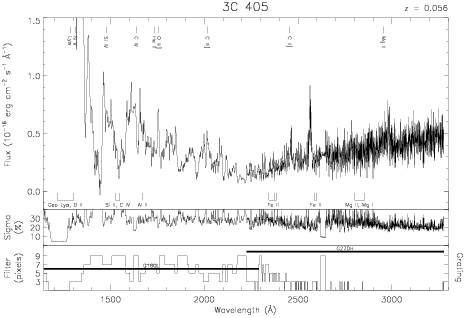Download dataset
TELESCOP= 'HST     '
INSTRUME= 'FOS     '
EQUINOX =               2000.0
DATE-OBS= '1993-05-23T14:56:31'
RA_PNT  =       299.8686250000
DEC_PNT =        40.7338722222
PA_PNT  =       189.3501552046
OBJECT  = '3C 405  '
ROOTNM01= 'Y1BF0102T'
GRNDMD01= 'SPECTROSCOPY'
DETECT01= 'BLUE    '
APERID01= 'A-1     '
FGWAID01= 'L15     '
ROOTNM02= 'Y1BF0103T'
GRNDMD02= 'SPECTROSCOPY'
DETECT02= 'BLUE    '
APERID02= 'A-1     '
FGWAID02= 'L15     '
ROOTNM03= 'Y1BF0104T'
GRNDMD03= 'SPECTROSCOPY'
DETECT03= 'BLUE    '
APERID03= 'A-1     '
FGWAID03= 'L15     '
ROOTNM04= 'Y1BF0105T'
GRNDMD04= 'SPECTROSCOPY'
DETECT04= 'AMBER   '
APERID04= 'A-1     '
FGWAID04= 'H27     '
ROOTNM05= 'Y1BF0106T'
GRNDMD05= 'SPECTROSCOPY'
DETECT05= 'AMBER   '
APERID05= 'A-1     '
FGWAID05= 'H27     '
ROOTNM06= 'Y1BF0107T'
GRNDMD06= 'SPECTROSCOPY'
DETECT06= 'AMBER   '
APERID06= 'A-1     '
FGWAID06= 'H27     '